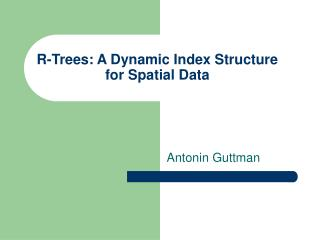Download PresentationR-Trees: A Dynamic Index Structure for Spatial Data

# R-Trees: A Dynamic Index Structure for Spatial Data - PowerPoint PPT PresentationDownload Presentation## R-Trees: A Dynamic Index Structure for Spatial Data

- - - - - - - - - - - - - - - - - - - - - - - - - - - E N D - - - - - - - - - - - - - - - - - - - - - - - - - - -
##### Presentation Transcript

1. R-Trees: A Dynamic Index Structure for Spatial Data Antonin Guttman

2. R-Tree: Why, What … ? • Why do we need R-Trees? • What are R-Trees? • How do I perform operations? • Alternatives? Why not a B+ tree?

3. Properties of R-Trees • Height Balanced • 2 types of nodes • Leaves point to disk pages • Records in the leaves point to actual data objects • For a max capacity of M, min occupancy should be M/2 • Completely dynamic • Guaranteed Fan-out of M/2 • Every leaf record is a smallest bounding box. • Root has at least two children

4. R-Trees: The Structure. • Internal nodes : ( rectangle, child pointer) • N dimensional rectangle. • Pointer to all rectangles that are cointained. • Leaf Nodes : (MBR , tuple-identifier) • MBR is minimum bounding rectangle • Tuple-identifier is a pointer to the data object.

5. R-tree of order 4

6. m n o p a c d e k l b f g h i j Example

7. m n o p a c d e k l b f g h i j m Example a b c d

8. m n o p a c d e k l b f g h i j n m Example e f a b c d

9. m n o p a c d e k l b f g h i j n m o p Example e f a b g h c d i

10. R-Trees: Operations • Inserts • Deletes • Updates ( delete and re-insert) • Queries/Searches • Names of all the roads in 1 sq km area? • Which buildings would be encountered between Roger’s Hall and Reitz Union? • Give me all rectangles that are contained in the input rectangle. • Give me all rectangles intersecting this rectangle.

11. Insert • Similar to insertion into B+-tree but may insert into any leaf; leaf splits in case capacity exceeded. • Which leaf to insert into? (Choose Leaf) • How to split a node? (Node Split)

12. n m o p Insert: Choose Leaf

13. Node Splitting • Quadratic method • Select max area gradient in the nodes as seeds. • Start clustering from the seeds • Linear method • Select seeds with max separation using max x, y • Randomly assign rectangles to seeds

14. Delete • Search for the rectangle • If the rectangle is found, remove it. • If the node is deficient, • Put the remaining entries in a re-insert queue. • Adjust the parent rectangle if needed. • Continue this till you reach the root. • Re-insert in such a way that all internal nodes remain above the leaf nodes. • Adjust the rectangles making them smaller. • Alternative sibling combination like a B-tree. • But re-insertion shows similar performance and is simple to implement.

15. Performance Tests • R-Trees in C under UNIX on VAX11/780 computer running on 2D data(1057) for 5 page sizes • Linear node split was better than quadratic as expected. • CPU time unchanged with page sizes, indicating that when one side became full all split algorithms simply put everything in the other side. • Delete is affected by the fill factor. • Search insensitive to the fill factor and split algorithm used. • Storage space is a function of the fill factor, page size and split algorithm • All split algorithms came in 10% of the best exhaustive search and split algorithm.

16. Performance: 2nd Innings • Same configuration but on various data sizes 1057, 2238, 3295 and 4559 rectangles. • Low CPU cost, close to 150 micro seconds. • Comparable performance of split algorithms • Most space was used by the leaf nodes

17. Conclusions from the paper. • R-Tree perform well for spatial data with non zero node sizes. • With smaller node structure can be used as an in-memory spatial data index. • CPU performance of in-memory R-tree index is comparable and there is no IO cost. • Linear split was almost as good as others. • It was fast. • Node split quality was a bit off-target, but it did not hurt the search performance noticeably. • Possible use with abstract data types and abstract indexes to streamline handling of spatial data.